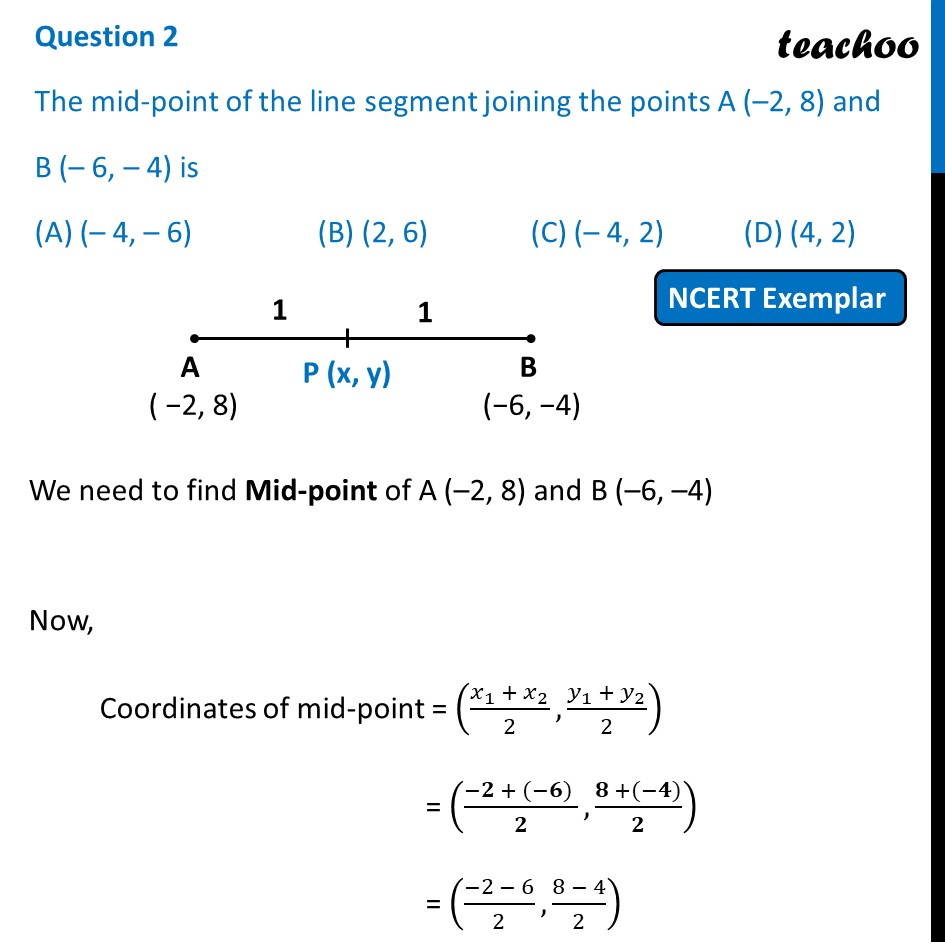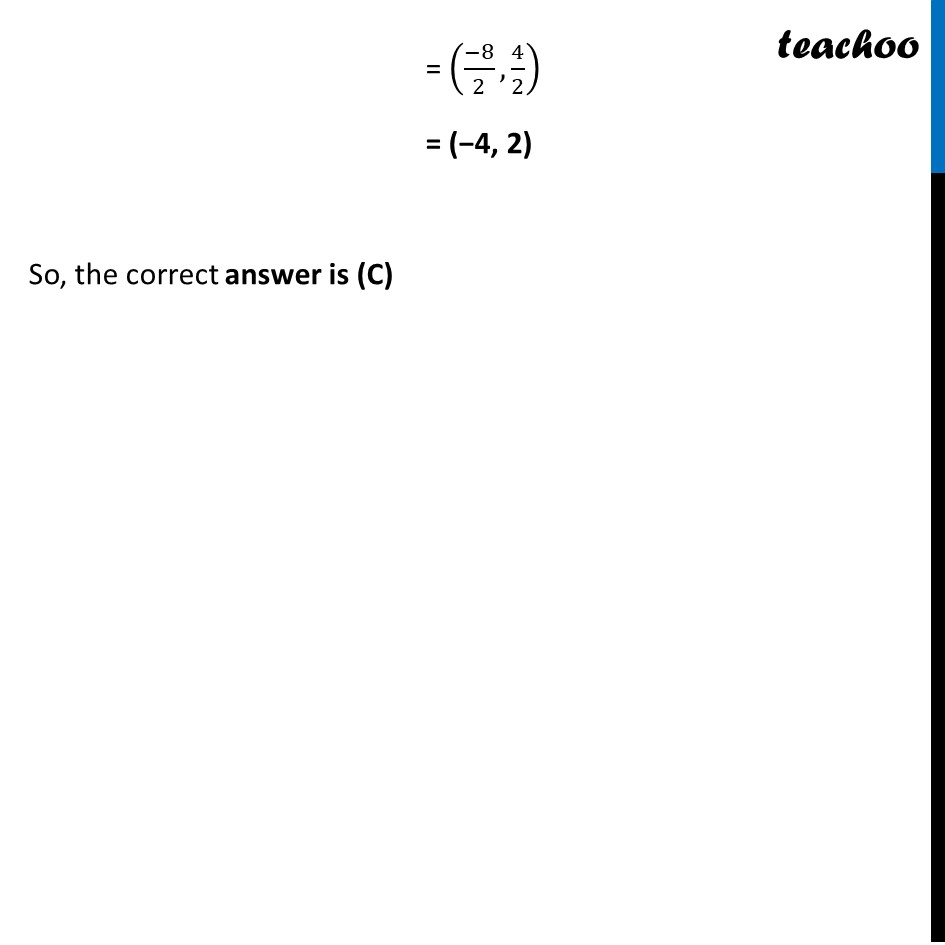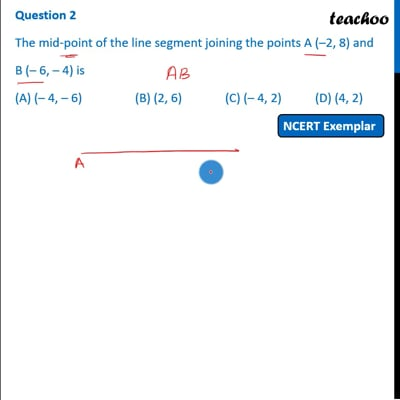NCERT Exemplar - MCQ

Chapter 7 Class 10 Coordinate Geometry
Serial order wise

## (D) (4, 2)This video is only available for Teachoo black users

Introducing your new favourite teacher - Teachoo Black, at only ₹83 per month

### Transcript

Question 2 The mid-point of the line segment joining the points A (–2, 8) and B (– 6, – 4) is (A) (– 4, – 6) (B) (2, 6) (C) (– 4, 2) (D) (4, 2) We need to find Mid-point of A (–2, 8) and B (–6, –4) Now, Coordinates of mid-point = ((𝑥_1 + 𝑥_2)/2,(𝑦_1 + 𝑦_2)/2) = ((−𝟐 + (−𝟔) )/𝟐,(𝟖 +(−𝟒))/𝟐) = ((−2 − 6)/2,(8 − 4)/2) = ((−8)/2,4/2) = (−4, 2) So, the correct answer is (C)